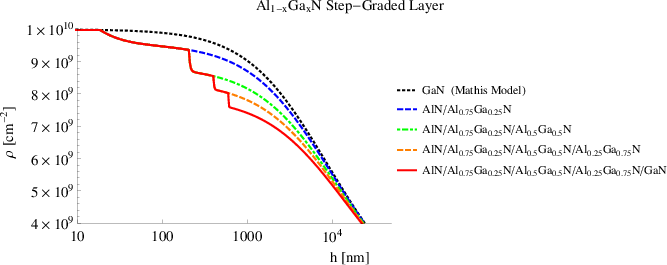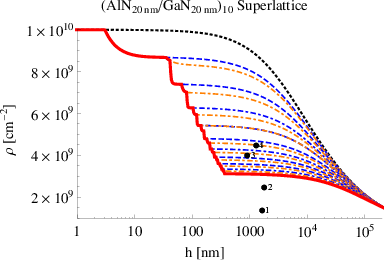### 5.4Threading dislocation density in heterostructures

In this section a model for the evolution of threading dislocations in GaN-based multilayers is derived by integrating Mathis’ model   with the model of Romanov  . The impact of the hexagonal symmetry is considered with an appropriate expression for the misfit dislocation density  [24,31]. In the first Paragraph 5.4.1 of this section, appropriate equations describing the motion of one isolated dislocation are derived. Then in Paragraph 5.4.2, the reaction-kinetic approach introduced by Romanov   is used to quantify the probability that two threading dislocations come in contact with each other. A model describing the threading dislocation density with increasing film thickness in multilayers is described through a set of differential equations (see Paragraph 5.4.3). These are then applied to a bilayer (Paragraph 5.4.5) in order to evaluate which parameters have the strongest impact on the results. After this, the threading dislocation density is calculated in a step-graded layer and superlattice to understand which structure is better for minimizing the threading dislocation population (Paragraph 5.4.6). Concluding the section, various design rules for dislocation filter are deduced and listed.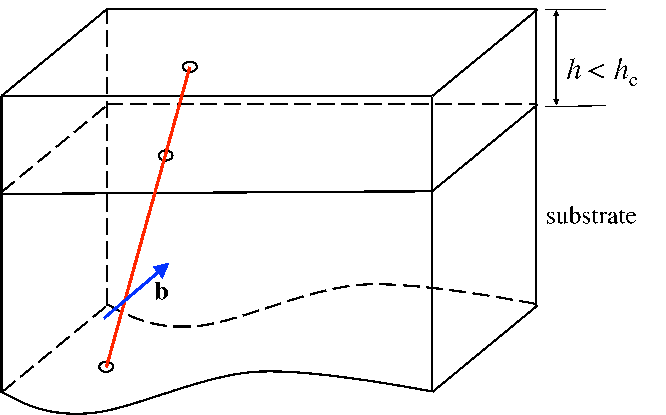(a)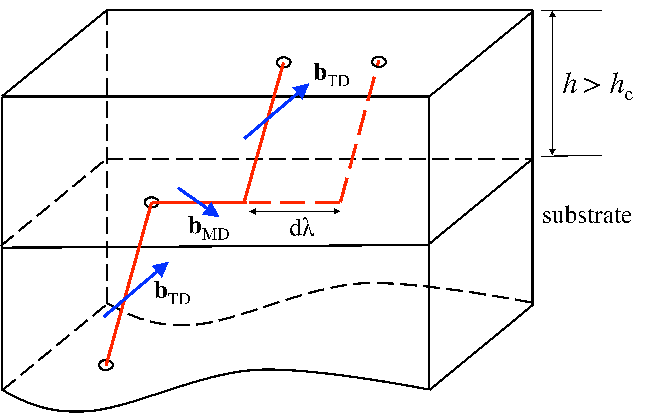(b)

 Figure 5.11: Basic processes of threading dislocation motion in a strained epitaxial film. 5.4 An isolated threading dislocation for h < hc for which no motion is possible. 5.4 Threading dislocation – misfit dislocation system for h > hc: increasing film thickness leads to an increasing configurational force on the threading dislocation which leads to threading dislocation motion and generation of additional misfit dislocation segment length.

#### 5.4.1Motion of an isolated TD

An isolated threading dislocation is depicted in Figure 5.12.Figure 5.12: Geometry of an isolated dislocation composed of a threading arm (TD) and a misfit segment (MD).

An uniformly strained layer of thickness hf is epitaxially bonded to a substrate of relatively larger thickness. The normal to the film-substrate interface is denoted by nf. The equibiaxial mismatch strain until critical thickness is denoted by ϵm. A threading dislocation is assumed to exist along a particular glide plane in the presence of the background mismatch strain field. The threading dislocation line extends from the free surface of the film to the substrate. The tangent vector of the threading dislocation is denoted l. During the film growth, it is assumed that the threading dislocation geometry remains self-similar. This means that the threading dislocation remains in the position of minimum energy with constant line direction l. The Burgers vector of the threading dislocation is denoted by b. The slip plane of the dislocation (the plane containing the dislocation line and the Burgers vector) is defined by its normal ng. The angle α between the dislocation line l and the normal nf of the free surface is calculated according to the minimization of the dislocation energy in Chapter 3. Therefore l can be derived from the relation: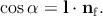(5.8)

ψ is defined as the angle between k and the projection of k to the film/substrate interface  :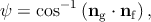(5.9)

where k is defined as(5.10)

The angle ω is defined as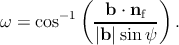(5.11)

After the vector k and the angle ω are calculated using the previous relations, the angle between b and the threading dislocation line l is denoted as θ, and can derived by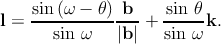(5.12)

During film growth, if film thickness is below the critical value, the threading dislocation is sessile, i.e., it is not able to glide. As a consequence, the threading dislocation does not move physically but the threading dislocation upper point moves along the free surface due to the inclination of the threading dislocation with respect to the growth direction. According to Figure 5.12, the point of intersection of the threading dislocation with the free surface of the film moves with direction f along the free surface. The velocity vS of the upper point of one sessile threading dislocation along the free surface is calculated as  :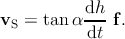(5.13)

As the film thickness increases after the critical thickness, the relaxation of the misfit stress occurs via the glide of the threading dislocation, which leaves behind one misfit segment along the interface. This misfit segment is generally called misfit dislocation and its length is denoted λ in this work. The misfit dislocation elongates until the complete relaxation of the misfit strain and the direction m of the elongation is the direction of the gliding threading dislocation  :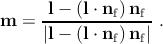(5.14)

In this work, the portion of the dislocation spanning the film thickness is still called the threading dislocation segment, or simply the threading dislocation. During the film growth after the critical thickness, λ increases as a result of relaxation of the initial misfit strain εm, causing a further glide of the threading segment. Concurrently, the threading dislocation length increases due to the increase of the film thickness. Thus after the critical thickness is reached, the threading dislocation intersection with the free surface will move not only due to the (eventual) inclination with respect to the free surface but also as a result of the threading dislocation glide, i.e., the misfit dislocation elongation along the interface. In other words, the upper point of the threading dislocation moves due to the inclination of the threading dislocation with respect to the free surface but also moves physically as a result of the threading dislocation glide along the interface. Therefore, the upper point of a glissile threading dislocation (i.e., a dislocation able to glide) has a velocity defined of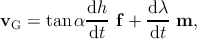(5.15)

where the first term takes the motion due to the inclination with respect to the film free surface into account, and the second term the motion due to the elongation of the misfit segment.

The elongation of the misfit segment is related to the relaxation of the structure caused by the film growth after the critical value. In order to establish a relation between the misfit dislocation elongation dλ and the increase of the film thickness dh, it is necessary to consider the expression for the misfit dislocation density (denoted as ρMD) reported in Section 5.3.

For the isotropic system, the differentiated form of expression (5.4) with respect to h is

 dρMD =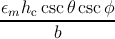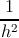dh = Ciso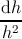. (5.16)
For the hexagonal system, the differentiated form of expression (5.6) with respect to h is
 dρMD =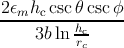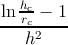dh = Chex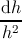. (5.17)
Misfit dislocations are generated through the motion of glissile threading dislocations, so it is possible to give a second definition of ρMD as a function of the total density ρG of the glissile threading dislocations: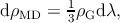(5.18)

where the factor 13 accounts for the use of a linear misfit dislocation density in a hexagonal grid array of misfit dislocations. When combining expression (5.16) or expression (5.17) with expression (5.18), and solving for dλ, the result is that dλ = Cdh∕(h2ρ G), with C = 3Ciso or C = 3Chex. Substituting the last relation in expression (5.15), the velocity of the glissile threading dislocation is expressed as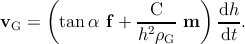(5.19)

#### 5.4.2Reactions among dislocations

Two threading dislocations with different Burgers vectors bi and bj and line directions li and lj are considered. The two threading dislocations can be either glissile or sessile. Supposing that the film thickness is increasing, the upper points of the two threading dislocations move along the film free surface until the point they come within a distance r such that the interaction forces are sufficient to initiate additional motion of dislocations. They can now start to react with each other. The driving force for the interaction between dislocations is the minimization of the internal energy. Three possible reactions (annihilation, fusion, and scattering) can occur depending on Frank’s criterion (see equation (5.3)). The interaction radius r within which all these reactions occur is intended to be the same value in this work  . The probability of reaction between these threading dislocations at any point on the free surface is supposed to be uniform and occur with a reaction rate of V ij. This is defined as a function of the difference between the velocities vi and vj of the threading dislocations  :(5.20)

The velocities are calculated properly for sessile and glissile threading dislocations through expressions (5.13) and (5.19), respectively.

Using 5.20 as well as the reaction kinetic approach introduced by Romanov  , it is possible to describe how the threading dislocation density changes during epitaxial growth. The densities of the i-th and j-th threading dislocation families are indicated with ρi and ρj respectively. If annihilation or fusion occur between dislocations of i-th and j-th kinds, then the diminishing density for the i-th kind can be calculated by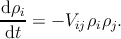(5.21)

and a similar expression can be written for the density of the j-th kind threading dislocations. When multiplying both the members of the previous equation by dt and combining with expressions (5.19) and (5.20), (5.21) becomes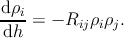(5.22)

where

 Rij = 2r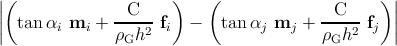. (5.23)
Fusion reactions between the i-th and j-th threading dislocations also have the effect of producing a new threading dislocation of the k-family; therefore a production term is taken into account for each kind of threading dislocation: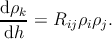(5.24)

Scattering reactions between the i-th and j-th threading dislocations can produce two k-th threading dislocations (see the reaction Table 5.1); therefore the previous equation is corrected as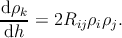(5.25)

In general, the evolution of density ρk of dislocations belonging to the k-th family with the film thickness is described by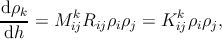(5.26)

where Mijk = 0 in case of annihilation, M ijk = 1 (ki and kj) for fusion and scattering reactions, and Mijk = 2 (ki and kj) for scattering reactions that produce two threading dislocations belonging to the same ρk family (see the reaction Table 5.1).

#### 5.4.3Sessile and glissile dislocations

The total density of threading dislocations, i.e., the number of threading dislocations per unit area of the free surface, is indicated with ρTD, the total density of sessile threading dislocations and the total density of glissile threading dislocations are indicated with ρS and ρG respectively. Therefore, below the critical thickness we have

 ρTD = ρS = ∑ k=120 ρ S,k. (5.27)
Above the critical thickness, it is assumed that each threading dislocation can glide, becoming glissile and leaving a misfit segment behind. Consequently, the total density of threading dislocations is the sum of sessile and glissile threading dislocation densities. If ρG indicates the total density of glissile threading dislocations, above the critical thickness this results in
 ρTD = ρG + ρS = ∑ k=120 ρ G,k + ∑ k=120 ρ S,k. (5.28)
Changing the film thickness by dh, the previous expression becomes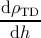= ∑ k=120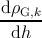+ ∑ k=120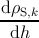. (5.29)
The complete relaxation of the structure stops the threading dislocation glide, and glissile threading dislocations become sessile again. Considering equation (5.19), the complete relaxation is reached when vG reduces to vS for h →∞.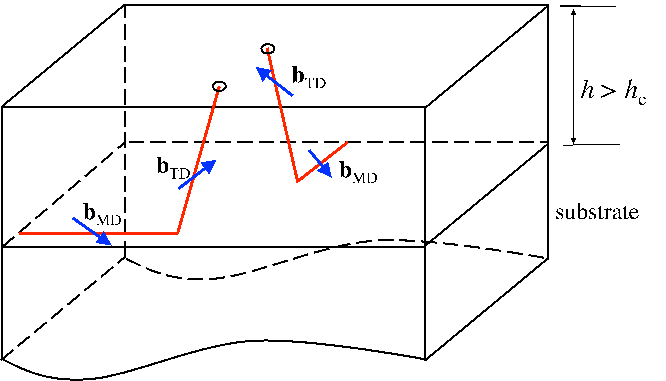(a) Initial configuration with two glissile threading dislocations in strained film of thickness hf > hc.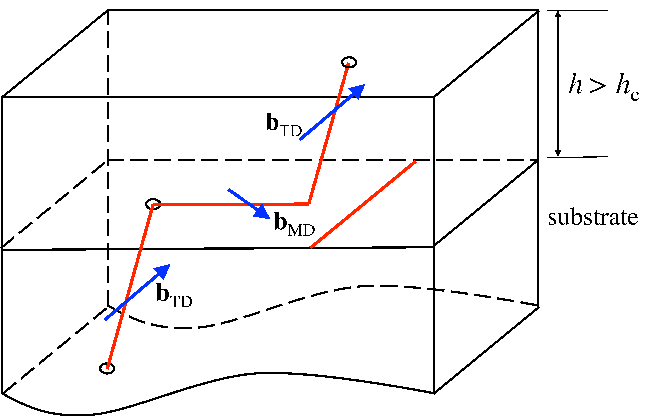(b) Blocking of threading dislocation motion by a misfit dislocation in the slip path of the threading dislocation.(c) Intermediate configuration with threading dislocations at the distance of the annihilation radius r.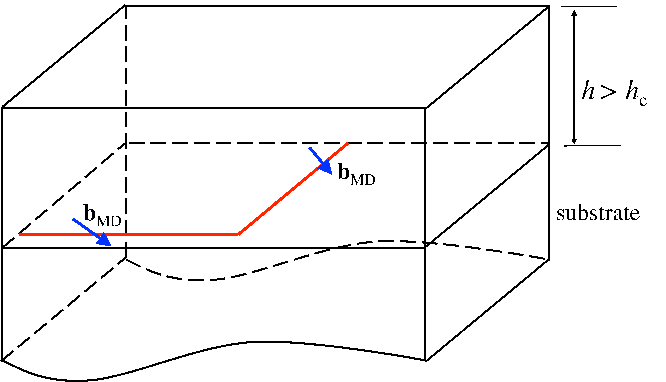(d) Annihilation of the two threading dislocations: final configuration with misfit dislocations only (the threading dislocations are annihilated).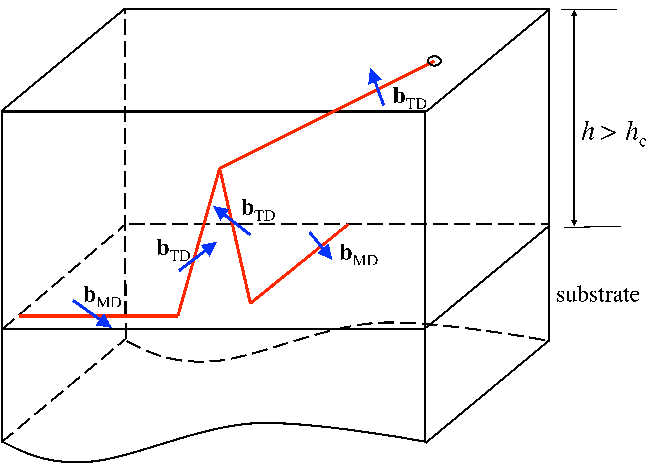(e) Fusion of the two threading dislocations: final configuration with one new threading dislocation.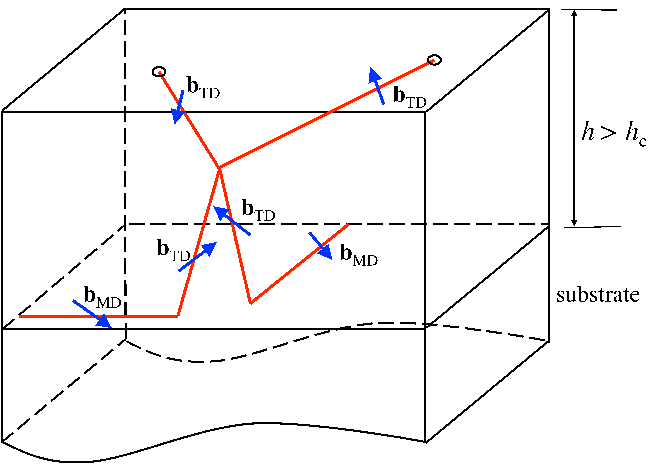(f) Scattering of the two threading dislocations: final configuration with two new threading dislocations.

 Figure 5.13: Movement and reactions of the threading dislocations

In parallel, other factors can stop the dislocation glide above the critical thickness and before the saturation point. During the motion, one glissile threading dislocation may be blocked by a misfit segment lying in the path of another moving threading dislocation, as shown schematically in Figure 5.4.3. The problem of threading dislocation blocking was treated in detail by Freund  . In the present treatment, once a threading dislocation has been blocked by a crossing misfit dislocation, its motion will be considered arrested for all further film growth. The chance of intersection of a randomly located glissile threading dislocation with a misfit dislocation is supposed to be ρG,kρMDdh. The result is a decrease of the density of k-type glissile threading dislocations by

 dρG,k = -ρG,kρMDdh, (5.30)
and consequently the density of the k-type sessile threading dislocations increases by
 dρS,k = ρG,kρMDdh. (5.31)
Also the reactions among threading dislocations can affect the ratio between glissile and sessile dislocations. The number of glissile dislocations decreases when two glissile threading dislocations annihilate each other and therefore the density of k-type glissile threading dislocations is decreased by the following:
 dρG,k = -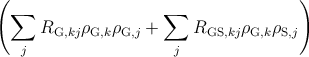dh. (5.32)
Annihilation is not the only reaction which decreases the density of glissile dislocations. Few experimental observations of the interaction between a glissile and a sessile dislocations are available in literature. Thus it is assumed in this work that the motion of one glissile dislocation ends when it encounters another threading dislocation (either glissile or sessile). At this point, the two threading dislocations react with each other by fusion or scattering and the resulting dislocation/dislocations remains/remain sessile. The density decreases by
 dρG,k = -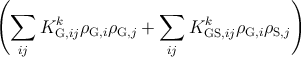dh. (5.33)
In parallel, the density of k-type sessile threading dislocations increases by
 dρS,k =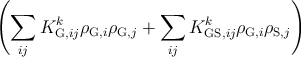dh. (5.34)
Based on equations (5.30), (5.32), and (5.33), by increasing the film thickness by dh, the total variation of the density of k-type glissile threading dislocations is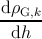= - ρG,k ρMD -∑ j RG,kj ρG,k ρG,j -∑ j RGS,kj ρG,k ρS,j -∑ ij KG,ijk ρ G,i ρG,j -∑ ij KGS,ijk ρ G,i ρS,j. (5.35)
The number of sessile threading dislocations is increased by the decrease of the glissile threading dislocation density (see equations (5.31) and (5.34)). In addition the reactions among sessile threading dislocations affect the density of the k-type sessile threading dislocation:
 dρS,k =dh. (5.36)
The k-type sessile threading dislocations can annihilate with glissile dislocations of an another family, therefore
 dρS,k = -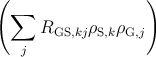dh. (5.37)
Based on equations (5.31), (5.34), (5.36), and (5.37), by increasing the film thickness by dh, the total variation of the density of k-type sessile threading dislocations is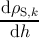= + ρG,k ρMD + ∑ ijKG,ijk ρ G,i ρG,j + ∑ ijKGS,ijk ρ G,i ρS,j + ∑ ijKS,ijk ρ S,i ρS,j -∑ jRS,kj ρS,k ρS,j -∑ jRGS,kj ρS,k ρG,j. (5.38)
The variation of ρTD with the film thickness dh is obtained by combining expression (5.29) with expressions (5.17), (5.35) and (5.38):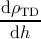= ∑ k=120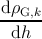+ ∑ k=120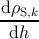, (5.39)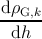= - ρG,k ρMD -∑ j RG,kj ρG,k ρG,j -∑ j RGS,kj ρG,k ρS,j -∑ ij KG,ijk ρ G,i ρG,j -∑ ij KGS,ijk ρ G,i ρS,j, (5.40)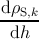= + ρG,k ρMD + ∑ ijKG,ijk ρ G,i ρG,j + ∑ ijKGS,ijk ρ G,i ρS,j + ∑ ijKS,ijk ρ S,i ρS,j -∑ jRS,kj ρS,k ρS,j -∑ jRGS,kj ρS,k ρG,j, (5.41)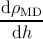=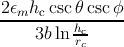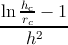dh =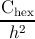. (5.42)
The calculation of the total density of the threading dislocation is performed with the software Wolfram Mathematica for the heterostructures considered in the next Paragraphs.

#### 5.4.4Initial and boundary conditions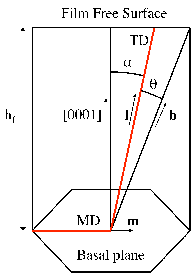Figure 5.14: Geometry of a mixed dislocation in GaN.

In this section the initial and boundary conditions used to solve the system of equations defined by equations (5.29), (5.17), (5.35) and (5.38) are listed.

• A single-crystal structure of Al1-xGaxN oriented along the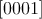direction is considered. The values of the lattice parameters of GaN and AlN are aGaN=3.22Å, cGaN=5.19Å, aAlN=3.11Å, and cAlN=4.98Å respectively. The values for the Al1-xGaxN alloy are calculated using Vegard’s law  .
• The core radius of each threading dislocation is supposed to be half of its Burgers vector.
• Anisotropic elasticity is used to calculate the critical thickness and the misfit dislocation density; consequently, C=Chex (see equation (5.17)).
• The interaction radius is 40nm  .
• The importance of the inclination angle for the calculation of the dislocation density was discussed in Section 5.2.1. The equilibrium values for the inclination angle were calculated in Section 3.7. According to this calculation, in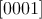GaN all types of threading dislocation are parallel to the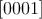direction. In reality, experimental observations show that this angle is influenced by many factors not considered in this work, such as temperature, island growth, doping, masking, and misfit stress relaxation. Mathis   and other authors   observed that a- and c-type threading dislocations are parallel to the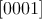direction but the (a + c) type threading dislocations are inclined by 12. Holec   showed how the island-growth mode can bend threading dislocations in the proximity of inclined facets. Cantu   grew Si doped Al0.49Ga0.51N films upon Al0.62Ga0.38N and observed inclined dislocations in the film. The inclination was suggested to be caused by the Si doping. Li   arrived at the same conclusion by doping a GaN-based film with Mg. In contrast to their explanations, Follstaedt   and coauthors realized that in similar structures, dislocations are bent before the introduction of any dopant. According to him, the relaxation of the misfit stress causes the bending. In this work, the screw and edge threading dislocations in GaN are supposed to be aligned with the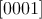direction, and the (a + c)-type threading dislocations inclined with respect to it (like assumed by Mathis  ). Based on these experimental findings, the inclination angle is assumed to be very small (5). The reason for this comes from evaluating the threading dislocation density in an unfavorable condition, i.e., the reaction probability is minimum.
• It is also assumed that the planar growth mode prevents threading dislocation inclination due to the vicinity with the inclined facets.
• The initial amount of threading dislocations is set at 1010cm-2 as reported by different authors  [38, 56, 81]. The initial threading dislocation density is composed of 70% a-type threading dislocation and 30% (a + c)-type threading dislocation. Different ratios are evaluated in Section 5.4.5.
• Several authors  [39, 49] show that c-type dislocations are not very mobile and not capable of reacting with other dislocations. Different explanations for this behavior have been proposed. The c-type dislocation oriented along the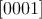direction is a screw dislocation. It might happen, for example, that screw dislocations exist at the center of a growth hillock or at the center of a nanopipe. In the case of a growth hillock, mixed dislocations must elongate and move across the hillock to reach the screw dislocation, making reactions less likely.
• A major assumption of the present model is a simplification of the geometry of the dislocation glide. It is assumed that a misfit segment and the upper point of a threading dislocation have the same direction, i.e., they have the same velocity f = m (see Figure 5.14), and equation (5.19) for glissile dislocations reduces to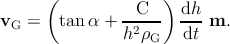(5.43)

As a consequence, it is assumed that only the (a + c)-type threading dislocations have the possibility to move, being that they are the only ones tilted with respect to the growth direction, the only ones to execute lateral motion toward each other and the only ones to react with an increase in the layer thickness. Despite this simplification, the model brings some insight and, although not quantitatively, at least qualitatively accounts for the contribution of glide to the threading dislocation density reduction.

#### 5.4.5GaN/AlN bilayer

The model described in Section 5.4 is used to calculate the threading dislocation density as a function of the film thickness hf in a  GaN film grown upon a AlN substrate. The results (red line in Figure 5.15) are compared with those from Mathis’ model   (blue line in Figure 5.15). The initial and boundary conditions are listed in Paragraph 5.4.4.

The development of the threading dislocation density according to Mathis can be divided into three stages, like as with semipolar bulk GaN (see Section 5.2.1). Initially, the density remains quite constant because no reactions occur due to the big distance among dislocations. After 1μm, the density decreases due to threading dislocation reactions. When all dislocations having reacted, their density assumes an asymptotical value, reaching the saturation point at 1mm.

The threading dislocation density according to the model proposed in this work is represented by the red solid line in Figure 5.15. This can be divided into five stages.

1.
Initially the density remains constant because dislocations are too far from each other to react.
2.
After the critical thickness is reached, which is 3μm according to Steeds  , dislocations glide along the interface and this causes the reactions. Therefore, the threading dislocation density decreases in the 10nm above the critical thickness. As a result of the threading dislocation glide, the density is 15% less with respect to what was predicted by Mathis.
3.
After hf = 15nm, the density is quite constant because dislocations not longer glide (since the misfit stress is totally relaxed) but reactions driven by threading dislocation inclination do not take place until 1μm.
4.
After hf = 1μm, reactions caused by threading dislocation inclination occur, which decreases the density until the point the model reduces to the Mathis model at hf = 10μm.
5.
For hf > 10μm, the behavior is equal to that of the Mathis model.

Experimental observations (from private discussions with Dr. Baumgartl) confirm that the presence of the interface and the subsequent glide of the dislocations decreases the threading dislocation density. Therefore, the improved model agrees better with the experimental observations respect to the Mathis’ model.

The red dotted line in Figure 5.15 represents the threading dislocation density – according to the model described in this work – without threading dislocation blocking caused by the presence of misfit dislocations along the interface. The red solid and dotted lines are close to each other meaning that threading dislocation blocking is negligible. This means that the first term from the left sides of both equations (5.35) and (5.38) is negligible.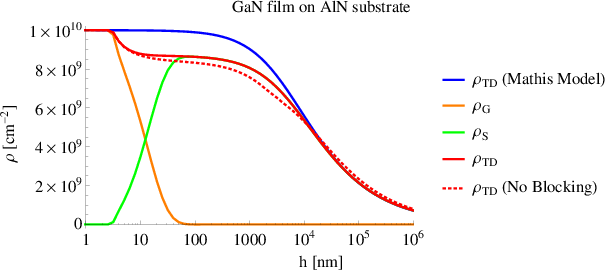Figure 5.15: The red solid line shows the threading dislocation density, calculated according to the model described in Section 5.4, as a function of the GaN film thickness h. The GaN film is grown upon an AlN thicker layer. The green and orange lines show the sessile and glissile threading dislocation densities, respectively. The red dotted line shows the threading dislocation density, according to the same model, without threading dislocation blocking. The blue line shows the threading dislocation density, calculated according to Mathis’ model  , as a function of the GaN film thickness h.

In the following, the threading dislocation density for the same bilayer – GaN film upon AlN substrate – is calculated, thus the initial conditions are changed.

One assumption is that only (a + c)-type threading dislocations are capable of gliding. In order to increase the impact of the glide, the initial amount of (a + c)-type threading dislocations is raised from 30% up to 60% and 90%. The related results are shown in Figure 5.16. When the initial threading dislocation density is composed of 90% of (a + c)-type threading dislocations, the final density is reduced by 30% with respect to the value given by Mathis’ model for hf < 2μm. Instead, for hf > 2μm, the variation of the initial amount of (a + c)-type threading dislocations has a negligible impact.

The effect of the inclination angle α is evaluated keeping the other initial and boundary conditions equal to those reported in Section 5.4.4. Both Mathis’ model and the improved one are used to calculate the threading dislocation density for different values of α. Figure 5.17 shows that the variation of α becomes remarkable in both models at higher thicknesses. At hf = 1μm, the threading dislocation density with α = 60 is halved with respect to the case with α = 5. The models confirm that increasing the inclination angle – by island growth, doping, and strain relaxation – drastically improves the quality of the crystalline structure.

In conclusion, the crystalline quality of GaN film grown upon an AlN thicker layer is improved by increasing the inclination angle rather than other factors. The dislocation glide decreases the threading dislocation density only by 15% with respect to what was predicted by Mathis’ model for bulk structures. The presence of one interface is ineffective in reducing the dislocation density. The main goal now is to evaluate the impact of the glide in a more complex heterostructure, with many interfaces along which threading dislocation glide occurs.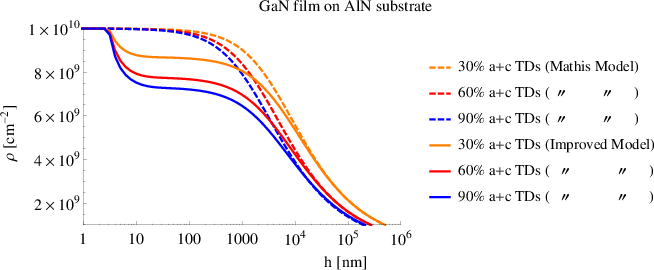Figure 5.16: Threading dislocation density as function of the GaN film thickness h calculated according to the Mathis model   and the treatment described in Section 5.4 with different initial amounts of (a + c)-type threading dislocations.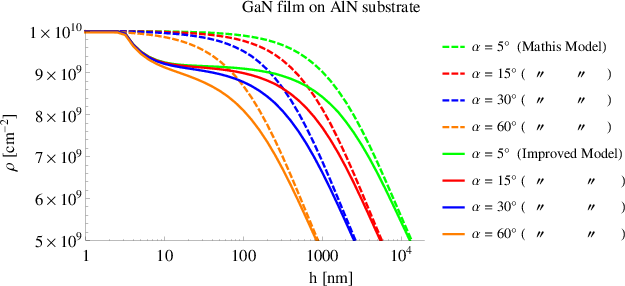Figure 5.17: Threading dislocation density as a function of the GaN film thickness h calculated according to the Mathis model   and the treatment described in Section 5.4 using different values for the inclination angle of the (a+c)-type threading dislocations.

#### 5.4.6GaN-based multilayer

The improved model described in Section 5.4 is used to calculate the threading dislocation density as a function of the multilayer thickness grown upon an AlN substrate. Two types of multilayer are considered. The first is a step-graded layer composed of Al0.75Ga0.25N (200nm) / Al0.5Ga0.5N (200nm) / Al0.25Ga0.75N (200nm) / GaN. The results are shown in Figure 5.18. The second structure is a (AlN/GaN)10 superlattice, and the related evolution of the threading dislocation density is shown in Figure 5.19. In both cases the initial and boundary conditions are the same as reported in Paragraph 5.4.4.

Considering the red line in Figure 5.18, the threading dislocation density after four interfaces at 1μm is 25% less than the initial value. A decrease of the threading dislocation density of technological relevance should be at least of one order of magnitude. Therefore this step graded layer seems to not be effective for improving the crystalline quality of the device.

As can be observed in Figure 5.18, the threading dislocation density quickly decreases suddenly after the critical thickness associated with each interface. This decrease is caused by threading dislocation glide and is proportional to the lattice mismatch associated with each interface.

The other curves of Figure 5.18 are related to multilayers with a lower number of layers with respect to the one which is represented by the red line. The results indicate that a lower number of layers decreases the threading dislocation density less.

Figure 5.19 shows the development of threading dislocation density in a (AlN/GaN)10 superlattice. This is composed of 10 periods of AlN/GaN. Each layer is 20nm thick. The superlattice is grown upon the AlN substrate and is h = 400nm high. A GaN layer extends for h > 400nm.